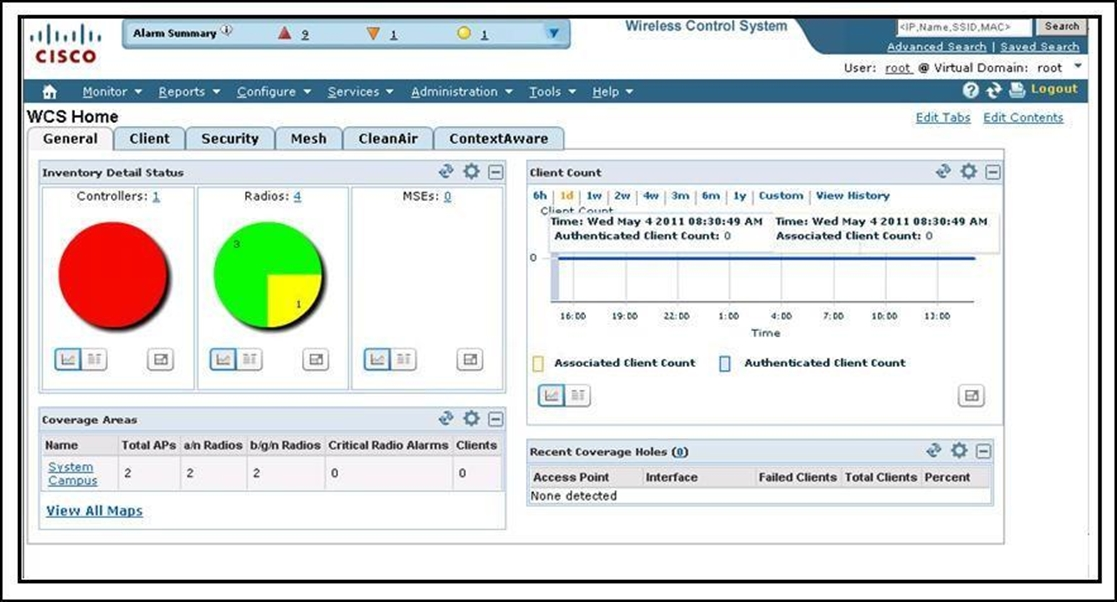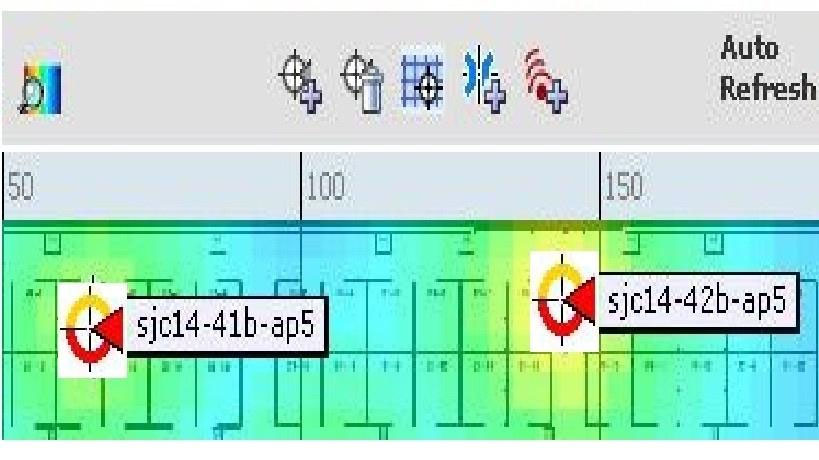# CCNA Wireless Implementing Cisco Wireless Network Fundamentals v1.0

Page:    1 / 40
Exam contains 605 questions

Refer to the exhibit.• B. Monitor
• C. Configure
• D. Services
• F. Tools

Explanation:
The Administration menu enables you to schedule tasks like making a backup, checking a device status, auditing your network, synchronizing the MSE, and so on. It also contains Logging to enable various logging modules and specify restart requirements. For user administration such as changing passwords, establishing groups, setting application security settings, and so on, choose AAA. From the Administration Menu, you can also access the licensing information, set user preferences, and establish high availability (a secondary backup device running WCS).
Reference:
http://www.cisco.com/c/en/us/td/docs/wireless/wcs/7-0/configuration/guide/WCS70cg/7_0wst.html#wp1060607

What is the equivalent of 26 dBm in milliwatts?

• A. 4 mW
• B. 40 mW
• C. 100 mW
• D. 400 mW
• E. 1000 mW

Explanation:
dBm = log10 (mW)*10
mW =10^(dBm/10)
Samples:
40 dBm=10.00 watts
36 dBm=4.00 watts
30 dBm=1.00 watts
27 dBm=500 milliwatts
26 dBm=400 milliwatts
Reference:
http://www.h-peters.com/dbmtomw.html

What is the EIRP value for a transmitter that has a transmitter capable of 15 dBm, an antenna that has a gain of 12 dBi, and a cable that connects the transmitter to the antenna that has 1 db loss?

• A. 26 dBm
• B. 100 mW
• C. 86 dBm
• D. 165 dBm
• E. 1000 mW

Explanation:
To determine EIRP follow this equation:
<Transmit Power> -Cable Loss + Antenna Gain = EIRP So in this case 15 - 1 + 12 = 26

Why are wireless analog video signals that are operating in the 2.4-GHz band particularly harmful to Wi-Fi service?

• A. Analog video is a strong signal and increases the SNR.
• B. Analog video is a constant signal with 100% duty cycle.
• C. Analog video signals are slow frequency hopping and tend to affect the entire band.
• D. Analog Video modulation is the same as Wi-Fi and causes interference.

Explanation:

What does the current European Telecommunications Standards Institute rule state is the 2.4-GHz maximum transmitter output power for point-to-point installations?

• A. 16 dBm
• B. 17 dBm
• C. 20 dBm
• D. 30 dBm
• E. 36 dBm

Explanation:

What are two attributes used to characterize antenna performance? (Choose two.)

• A. attenuation
• B. beamwidth
• C. gain
• D. harmony
• E. interference

Explanation:

Refer to the exhibit.Which two statements about the status of the respective AP on a Cisco WCS version 7.0 map does this icon represent? (Choose two.)

• B. The 802.11a/n radio has a minor fault.
• C. The 802.11a/n radio has a major fault.
• E. The 802.11b/g/n radio has a minor fault.
• F. The 802.11b/g/n radio has a major fault.

Explanation:
The icon with the top half yellow and the lower half red indicates that the optional 802.11a Cisco Radio (top) has a minor fault, and the 802.11b/g Cisco Radio
(bottom) has a major or critical fault. The worst of the two Cisco Radio colors determines the color of the large triangular pointer. http://www.cisco.com/c/en/us/td/docs/wireless/wcs/7-0MR1/configuration/guide/WCS70MR1/maps.html#wp1090761

Which modulation technique allows you to achieve a data rate of 54Mb/s in the 2.4GHz-band?

• A. Complimentary Code Keying
• B. Differential Binary Phase Shift Keying
• C. Differential Quadrature Phase Shift Keying

Explanation:

Which description best describes upfade in a multipath environment?

• A. In this situation, multiple signal paths are not sent at exactly the same time. The receiver receives a positive crest on the primary signal and a negative crest on the secondary signal.
• B. In this situation, the multiple signal paths are distorted and difficult to understand.
• C. In this situation, the multiple signal paths are weaker than they should be because the signals are out of phase with each other.
• D. In this situation, the signal is stronger than it should be because multiple signal paths are received twice at exactly the same time, which results in the multiple signals being in-phase.

Explanation:
If a signal is received twice at exactly the same time, the secondary wave adds its power to the primary wave, so the receiver gets twice the positive energy
(positive crest) at the same instant, then twice the negative energy (negative crest) at the same instant. The result is that both waves add up to twice the amplitude
(energy) of a single wave, and both signals are said to be in phase (both signals are said to have an angle of 0 degrees). This rare condition is called upfade. If the second signal negative energy (negative crest) reaches the receiver just when the first signal positive energy (positive crest) also reaches the receiver, both signals can cancel each other, resulting in no signal at all (this is the principle used in noise cancellation headsets).

Which two factors must be considered when evaluating an RF interferer for severity? (Choose two.)

• A. distance from the AP
• B. dBm
• C. the type of security crack being used
• D. duty cycle
• E. number of interfering IP stations in the cell
• F. duplicate SSID

Explanation:
On controller code 7.0 and later, you can configure Event Driven RRM (EDRRM) from the Wireless >802.11a/n [802.11b/g/n] > RRM > DCA page. Enabling
EDRRM allows the controller to bypass the 600-second default interval for DCA and change an AP channel immediately if a disturbing non-802.11 interference is detected on the AP channel. You can set the sensitivity threshold to High (60), Medium (50), or Low (35). This threshold refers to the Air Quality index. CleanAir- capable APs (3500 and 3600 Series APs, with their CleanAir capable chipset) can detect and identify non-802.11 interferences and report them to the controller.
These APs can report interferers when in local mode and monitor mode. Notice that the AP cannot report to the controller when in Spectrum Only Monitor Mode
(SOMM). The controller uses the reported information to list non- 802.11 interferers along with their zone of impact and severity and establish an Air Quality Index
(AQI) from 100 (perfect) to 0 (network unusable). Notice that the logic of the global AQ index (higher is better) is exactly the opposite of the logic of the individual interferer severity index (higher is more severe, and therefore worse). The severity index is calculated individually by each detecting AP based on the power
(signal strength), duty cycle (how often the device uses the air per second), and detected type of interferer. The louder an interferer device, the higher the duty cycle will mean a higher severity index for the reporting AP. The severity index is calculated for a specific device, without regard to what channels are being affected.

What unit of measurement is used to represent the strength of an antenna's radiation pattern?

• A. dBi
• B. dBm
• C. mW
• D. GHz

Explanation:

How many dBm is 40 mW?

• A. 10 dBm
• B. 16 dBm
• C. 20 dBm
• D. 22 dBm
• E. 40 dBm

Explanation:
The dB measures the power of a signal as a function of its ratio to another standardized value. The abbreviation dB is often combined with other abbreviations in order to represent the values that are compared. Here are two examples:
You can calculate the power in dBs from this formula:
Power (in dB) = 10 * log10 (Signal/Reference)
This list defines the terms in the formula:
Here is an example. If you want to calculate the power in dB of 50 mW, apply the formula in order to get:
Power (in dB) = 10 * log10 (50/1) = 10 * log10 (50) = 10 * 1.7 = 17 dBm
Because decibels are ratios that compare two power levels, you can use simple math in order to manipulate the ratios for the design and assembly of networks.
For example, you can apply this basic rule in order to calculate logarithms of large numbers: log10 (A*B) = log10(A) + log10(B)
If you use the formula above, you can calculate the power of 50 mW in dBs in this way:
Power (in dB) = 10 * log10 (50) = 10 * log10 (5 * 10) = (10 * log10 (5)) + (10 * log10(10)) = 7 + 10 = 17 dBm http://www.cisco.com/c/en/us/support/docs/wireless- mobility/wireless-lan-wlan/23231-powervalues-23231.html

Which calculation computes the EIRP of an antenna?

• A. EIRP = Tx power (dBm) + Antenna Gain (dBi) - Cable Loss (dB)
• B. EIRP= Cable Loss (dB)+ Antenna Gain (dBi) - Tx power (dBm)
• C. EIRP = Cable Loss (dB)+ Antenna Gain (dBi) / Tx power (dBm)
• D. EIRP = Tx power (dBm) + Antenna Gain (dBi) / Cable Loss (dB)
• E. EIRP = Antenna Gain (dBi) - Cable Loss (dB) * Tx power (dBm)
• F. EIRP = Tx power (dBm) * Antenna Gain (dBi) / Cable Loss (dB)

Explanation:
EIRP (Effective Isotropic Radiated Power) is the actual amount of signal leaving the antenna and is a value measured in db and is based on 3 values:
-> Transmit Power (dBm)
-> Cable Loss (dB)
-> Antenna Gain (dBi)
The dB measures the power of a signal as a function of its ratio to another standardized value. The abbreviation dB is often combined with other abbreviations in order to represent the values that are compared. Here are two examples: dBm --The dB value is compared to 1 mW. dBw --The dB value is compared to 1 W.
You can calculate the power in dBs from this formula:
Power (in dB) = 10 * log10 (Signal/Reference)
This list defines the terms in the formula:
log10 is logarithm base 10.
Signal is the power of the signal (for example, 50 mW). Reference is the reference power (for example, 1 mW).

How to find EIRP -
To determine EIRP follow this equation:
<Transmit Power> -Cable Loss + Antenna Gain = EIRP

Effective Isotropic Radiated Power is calculated by using which three values? (Choose three.)

• A. antenna bandwidth
• B. antenna gain
• C. cable loss
• E. SSID
• F. transmission power

Explanation:

What increases bandwidth and resists multipath problems by carrying data in subcarriers?

• A. Direct Sequence Spread Spectrum
• B. Frequency Hopping Spread Spectrum
• C. Narrow Band Frequency
• D. Orthogonal Frequency Division Multiplexing

Explanation:

Page:    1 / 40
Exam contains 605 questions